# Lecture 36: Diffraction on multiple slits

## Interference pattern of the light diffracted on multiple slits

• In technology, of immense significance are interference patterns that are formed after diffraction on multiple parallel slits.
 For one, this is the main technique to split the light into spectral components according to the wavelengths used in modern spectrographs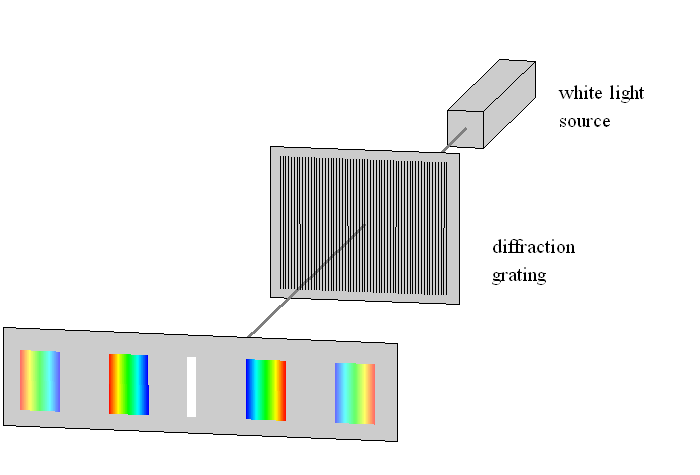What is the distribution of the electric field on the screen between diffraction grating that consists of N parallel slits, each of width a and separated by distance d ?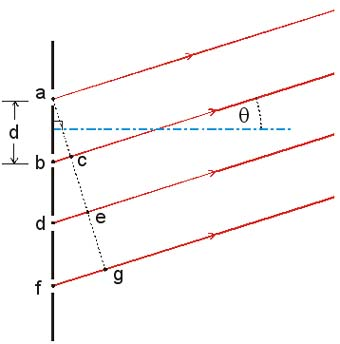## Calculation of the intensity distribution in diffraction grating

• It is rather easy again to calculate (but see textbook for low math discussion) in far-field Fraunhofer regime
• We have learned that a single slit in far field regime creates the distribution of the electric field given by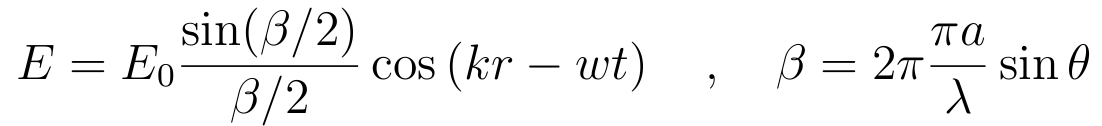• The total electric field from N slits in a grating is just the sum of individual contributions
• Because the sources are coherent, this sum results in interference of individual contributions.
• We must take into account relative phases of the contributions from individual slits to a point on screen. The formula above was written so that we took the phase of the light that passes through the center of our single slit to be zero. Then each subsequent slit has an additional phase shift due to light path length difference. So the contribution of individual slit number i should be written as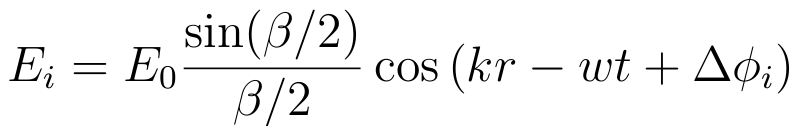and the total is just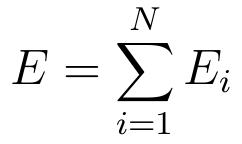• We shall use the following setup: we consider even number of slits, with a first pair of slits ( a and b ) at distances ± d/2 from the central line, the second pair at distances ± 3d/2 and so on.
• The phase difference between paths from the centers of slits and the central line are, pairwise
Δφ1 = ± φ/2 , Δφ2 = ± 3 φ/2 , … , Δφp = ± (p-½) φ          &phi ≡ 2 &pi (d/λ) sinθ
where p enumerates the pairs and φ is the phase difference in two-slit interference experiment.
• Now to sum over all slits, we sum the contributions of two slits in each pair, and then sum over all the pairs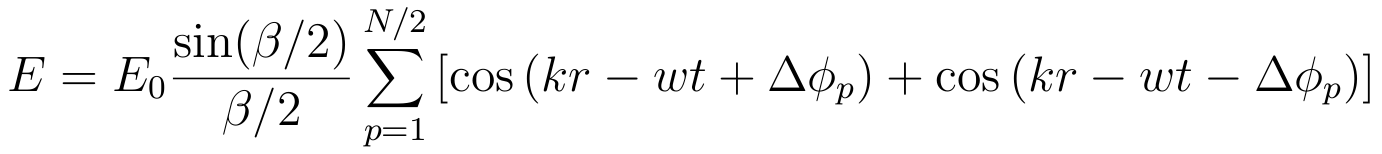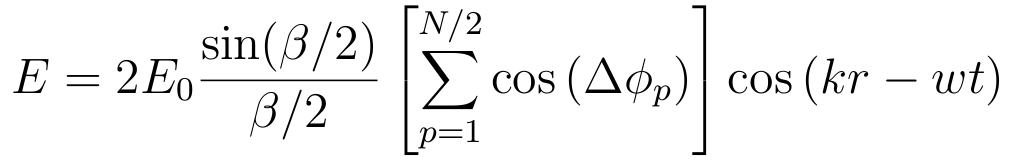• So for intensity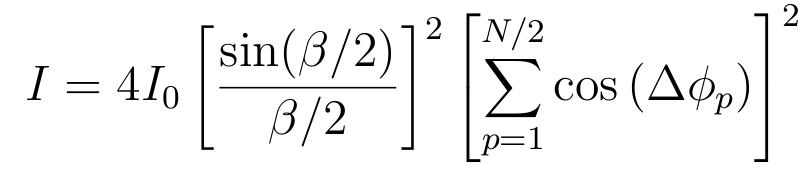• Or plugging everything in
 N slits: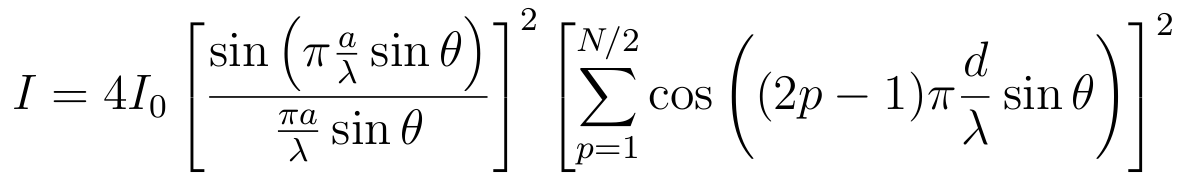## Was it tough? But now you know everything about diffraction grating ! Let's investigate the result. There are lots of interesting conclusions to be made

• The interference pattern from the diffraction grating is just the production of the diffraction pattern from a single slit of width "a" and interference pattern from multiple very narrow slits
• The first effect is determined by the phase factor β ≡ 2 π a/λ sinθ
• The second effect is determined by the phase φ ≡ 2 π d/λ sinθ
• Indeed, in case of just two slits (N=2), we recognize both factors right away

 Two slits: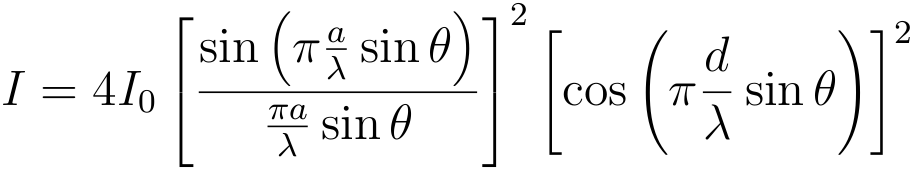Double slit intensity pattern, d > a (on the plot the width a is marked as b). Single slit diffraction factor oscillates with lesser frequency and modulates the higher frequency multi-slit interference factor, which determines maxima and minima of intensity.• Central peak of intensity at θ=0 in diffraction grating with N slits is N2 times the central intensity from individual slit

## Diffraction grating with large number of narrow slits

• Such gratings are used widely in spectroscopy.
• They can be manufactured very differently - "painted" sequence of dark lines on the glass, scratches on the glass to use reflected rather than transmitted light. So slits can be called rulings or lines.
• They are typically characterized by the number of slits per unit length , for example 2000 per cm. In this case one needs to compute the separation d between the slits.
• For such gratings, diffraction on individual slit is not that important, we focus on interference factor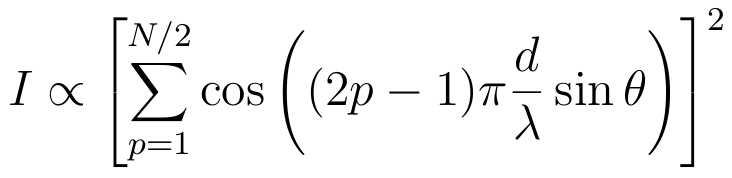This pattern has maxima where all cosine terms are either 1, or all are -1 . All cosines are +1, when &pi (d/λ) sinθ = 2 m π All cosines are -1, when &pi (d/λ) sinθ = (2 m + 1)π Hence, any integer number of π will do, and the directions of intensity maxima in pattern from diffraction grating are sinθm = m λ/d i.e. exactly the same as for two-slit interference. The value of m is called the order of the maximum .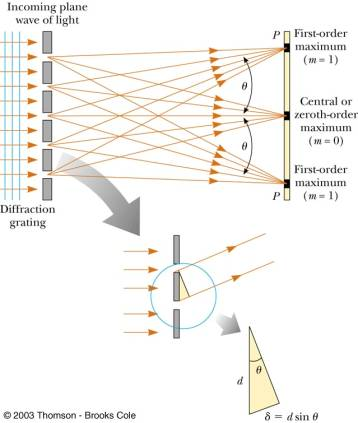• So if positions of the maxima are the same for all N (neglecting diffraction on a slit), what those multiple slits are for ?
• First of all, intensity at the peaks increases as N2
• But more importantly, increasing number of slits decreases the width of the peaks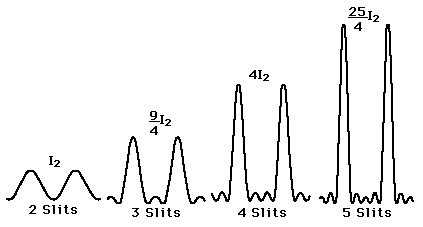## Why do the maxima become narrower with more slits in the grating and why is this important in practice ?

• In detail we are dealing with properties of Fourier transform. Without details, the width of the maxima is determined by the fastest oscillating term in the expression for intensity.
cos( (N-1) π (d/λ) sinθ )
• It changes from 1 at the peak to 0 when the cos argument changes by π/2 . This gives you half of the width of the peak. Hence, the width
Δ sinθ ≈ λ/(N d)
• In other words, as we see from the graph above, the number of minima between to main maxima is N-1
• Narrower the maxima, better we can distinguish the neighbouring ones. This plays a critical role in improving chromatic resolution of the spectrographs.

## Spectrographs and their chromatic resolving power (resolution)

• Spectrograph is a device to decompose the light into its spectral components and to measure relative intensities of different spectral components,obtaining the spectrum of the light.  Modern spectrograph utilize diffraction gratings of different construction to achieve this result. Its basic optical scheme looks like in the figure to the right.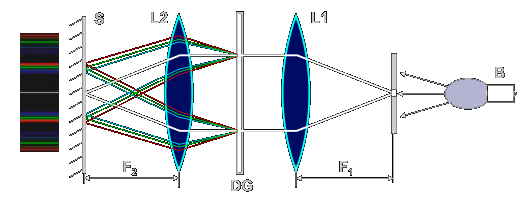• The idea is simple - the light of different wavelength after passing through diffraction grating will have peaks of intensity at different angles
θm(λ) ≈ m λ/d
producing the image something like this one for atomic hydrogen emission.  Notice that the zero order, m = 0 , maxima for all wavelengths coincide, there is no spectral decomposition. First order, m = 1 , maxima at different wavelength are separated, but overlap, one can say are not very well resolved At second order, m = 2 , the maxima are well separated i.e well resolved The redder light is further from the center than the bluer light.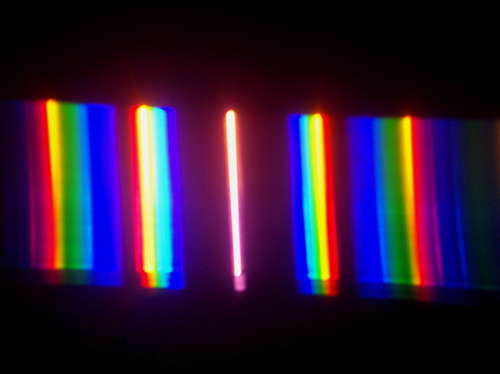• Let us understand the spectral resolution in more detail
• The angular separation between maxima of the same order at wavelengths λ and Δλ
Δλθm = ( m/d ) Δλ
• But the width of the maxima is
Δ θ ≈ λ/(N d)
• Rule-of-thumb criterium is that we resolve two spectral lines when they are separated by the angle that exceeds the width of the line
Δλθm > Δ θ
• This tells us how different should be the two wavelengths to be resolved
Δ λ/λ > 1/(m N)
• Chromatic resolving power of the spectrograph is defined as R ≡ λ/Δλ = m N is improved with increase of the number of slits in the grating and with utilizing spectra of higher order
• It is a matter of design to choose slit width a, slit separation d and number of slits N
• Smaller a, less diffraction pattern from the individual slit modulates the spectrum
• Smaller d, wider the angle to the maximum, but only lower order spectra are available, which may affect chromatic resolution.
• Larger N , higher the resolution and intensity, but more difficult to manufacture and larger the grating is.
• etc.